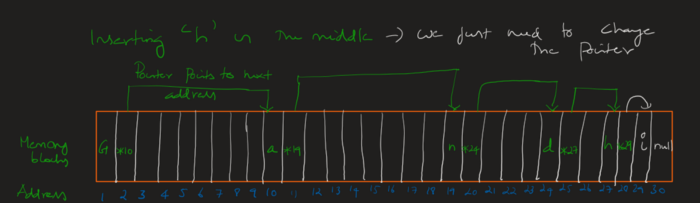top of page

# Data structures from scratch- Bot-up series #9[Linked List]

Let us recap what we have learned so far

We start storing values in a contiguous memory location.

Why? Because we can easily access it

For example, If a value stored occupies 4 bytes each, we easily locate all the elements in the array

How? The first element starts at 0, second at 4, third at 8 and so on.

Elements can be found by simple formula 4*n where n->Position

This gives easy accessibility at any random position.

First, we simply stored in a contiguous memory location and then we have space where we didn’t get continuous slots in a single go. We introduced the concept of ‘pointers’ where addresses are stored contiguously.

Then we encountered a length problem because it is not always possible to announce the length of the array in advance.

Then we introduced ‘Dynamic Array’ where allocation is taken care of by the system itself(Programming language)

Note: Dynamic involves movement.

Therefore,

Continuous memory problem → Pointers(This is also a static array). Announcement of length problem →Dynamic arrays.

Let’s marry these two in order to get the full benefit

But before that, we need to understand the cons of array

Mainly insertion and deletion of elements in arrays are difficult even though accessibility is faster

Why? If we want to insert the element

Case 1:Inserting at the end →No problem

Case 2:Inserting at the middle →Indexes of all the elements which come after new element changes

Case 3:Inserting at start →All indexes change

If the index changes, we need to move the elements and this takes time

This introduces us ‘Time cost’ in the array while inserting and deleting

Therefore we will build a data structure that can insert or delete elements easily

For that, we will borrow the pointer concept and dynamicity(No length announcement) from arrays

For that, we need two slots

One for storing value and the other for the pointer.In this case, the pointer always points to the next value’s memory address

The first value won’t be addressed by the pointer and it is called Head

The last value will point to ‘null’ and it is called Tail

The combination of value and pointer is called ‘Node’

There are many things hidden in plain sight here

1)We can store wherever we want

2)It can be of any size because any number of values can be linked by pointer

3)Insertion and deletion only requires rearranging of before and after pointers of the particular element concerned.Well, there is a name to the structure we built

Yes, this is called ‘Linked list’